+250
2.5 to 1Tom Brady
22.3% implied probability

+450
4.5 to 1Patrick Mahomes
14.2% implied probability

+750
7.5 to 1Matthew Stafford
9.2% implied probability

+750
7.5 to 1Dak Prescott
9.2% implied probability

+800
8 to 1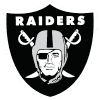Derek Carr
8.7% implied probability

+1000
10 to 1Justin Herbert
7.1% implied probability

+1800
18 to 1Kyler Murray
4.1% implied probability

+2800
28 to 1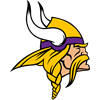Kirk Cousins
2.7% implied probability

+4000
40 to 1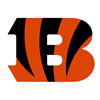Joe Burrow
1.9% implied probability

+4000
40 to 1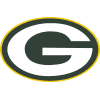Aaron Rodgers
1.9% implied probability

+4000
40 to 1Sam Darnold
1.9% implied probability

+5000
50 to 1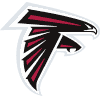Matt Ryan
1.5% implied probability

+6500
65 to 1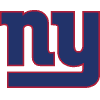Daniel Jones
1.2% implied probability

+6500
65 to 1Ben Roethlisberger
1.2% implied probability

+8000
80 to 1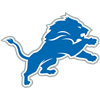Jared Goff
1.0% implied probability

+10000
100 to 1Mac Jones
0.8% implied probability

+10000
100 to 1Trevor Lawrence
0.8% implied probability

+10000
100 to 1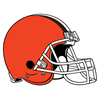Baker Mayfield
0.8% implied probability

+10000
100 to 1Jalen Hurts
0.8% implied probability

+10000
100 to 1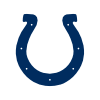Carson Wentz
0.8% implied probability

+10000
100 to 1Taylor Heinicke
0.8% implied probability

+10000
100 to 1Jameis Winston
0.8% implied probability

+10000
100 to 1Jimmy Garoppolo
0.8% implied probability

+10000
100 to 1Teddy Bridgewater
0.8% implied probability

+10000
100 to 1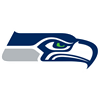Russell Wilson
0.8% implied probability

+10000
100 to 1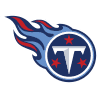Ryan Tannehill
0.8% implied probability

+15000
150 to 1Drew Lock
0.5% implied probability

+15000
150 to 1Zach Wilson
0.5% implied probability

+20000
200 to 1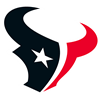Tyrod Taylor
0.4% implied probability

+20000
200 to 1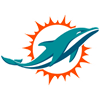Tua Tagovailoa
0.4% implied probability

+20000
200 to 1Trey Lance
0.4% implied probability

+25000
250 to 1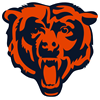Andy Dalton
0.3% implied probability

+25000
250 to 1Ryan Fitzpatrick
0.3% implied probability

+25000
250 to 1Justin Fields
0.3% implied probability

+50000
500 to 1Taysom Hill
0.2% implied probability